# Metric Deltas

## Computing Metric Deltas​

A metric delta refers to the difference in metric values between the test and control groups. This is usually the impact we care about when looking at experiment results. To account for the different number of users (or units) that consitute each group, we compare the mean metric value per user, not the total.

Two different metric deltas are available in Pulse. The absolute delta is simply the difference between the two means: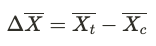It's often helpful to understand the impact relative to the baseline value of the metric. For example, an absolute delta of +1 clicks/user has different meanings with a baseline value of 1 (+100% increase) vs. a baseline value of 100 (+1% increase). The relative delta is computed using the mean of the control group as the baseline: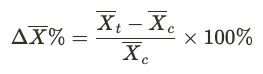## Computing Means​

Properly computing the groups means is critical for obatined meaningful metric deltas. The exact methodology for calculating the metric means depends on the type of metric.

### Event Count and Sum Metrics​

These metrics represent totals: Number of times an event occurs, sum of time spent, total purchase amount, etc. The mean is the average user-level total during the time period of the analysis.

The mean value of the metric X is given by: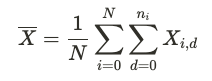where

• N is the number of users in the group
• ni is the number of days during the analysis period that user i was the experiment
• Xi,d is the metric value for user i on day d

Note that only user metrics recorded after a user has been exposed to the experiment are included in the group mean.

### Event DAU and User Accounting Metrics​

Event DAU metrics capture the number of distinct users that have the event each day. In Pulse results, they are normalized by the number of days the user has been in the experiment. This represents the probaility that a user is daily active for that event, i.e. the daily participation rate. In the terms defined above, the event DAU group mean is given by: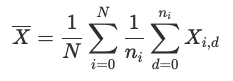The following user accounting metrics are computed in the same may: DAU, WAU, MAU_28day, L7, L14, L28

For new user accounting (new_DAU, new_WAU, new_MAU_28day) we count users that are new xAU at some point during the analysis window. So the group mean is given by:Where max(Xi) is the maximum value of the new xAU metric for user i.

### Custom Ratios, Means, Retention and Stickiness Metrics​

These are metrics such as click through rate, averge purchase value, sessions per user, etc. They're obtained by diving a numerator value, X, by a denominator value, Y. The mean value of a ratio metric R for an experiment group is given by: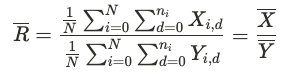Where N is the number of users in the experiment group that participate in the metric, i.e. have a non-zero denominator value. Xi,d and Yi,d are the X and Y values for user i on day d.

Different approaches exist for dealing with ratio metrics in experiments. This implementation was selected because it's statistically sound as well as interpretable, given that:

• R is the ratio of two means of independent observations: A set of user-level X values and a set of user-level Y values. This means the central limit theorem can be used to separately obtain the summary statistics of X and Y.
• The group means are computed in the same way as the topline metric value, making it easier to interpret the means and relate them to the topline metric.